• 详细来讲讲梯度下降算法原理，感受数学和程序的魅力吧！！
博文目录1. 概述2. 梯度下降算法2.1 场景假设2.2 梯度下降2.2.1 微分2.2.2 梯度2.3 数学解释2.3.1 α2.3.2 梯度要乘以一个负号3. 实例3.1 单变量函数的梯度下降3.2 多变量函数的梯度下降4. 代码实现4. 1 场景分析4. 2 代码5. 小结
1. 概述
本文将从一个下山的场景开始，先提出梯度下降算法的基本思想，进而从数学上解释梯度下降算法的原理，解释为什么要用梯度，最后实现一个简单的梯度下降算法的实例！
2. 梯度下降算法
2.1 场景假设
梯度下降法的基本思想可以类比为一个下山的过程。
假设这样一个场景：一个人被困在山上，需要从山上下来(找到山的最低点，也就是山谷)。但此时山上的浓雾很大，导致可视度很低；因此，下山的路径就无法确定，必须利用自己周围的信息一步一步地找到下山的路。这个时候，便可利用梯度下降算法来帮助自己下山。怎么做呢，首先以他当前的所处的位置为基准，寻找这个位置最陡峭的地方，然后朝着下降方向走一步，然后又继续以当前位置为基准，再找最陡峭的地方，再走直到最后到达最低处；同理上山也是如此，只是这时候就变成梯度上升算法了2.2 梯度下降
梯度下降的基本过程就和下山的场景很类似。
首先，我们有一个可微分的函数。这个函数就代表着一座山。我们的目标就是找到这个函数的最小值，也就是山底。根据之前的场景假设，最快的下山的方式就是找到当前位置最陡峭的方向，然后沿着此方向向下走，对应到函数中，就是找到给定点的梯度 ，然后朝着梯度相反的方向，就能让函数值下降的最快！因为梯度的方向就是函数之变化最快的方向(在后面会详细解释)
所以，我们重复利用这个方法，反复求取梯度，最后就能到达局部的最小值，这就类似于我们下山的过程。而求取梯度就确定了最陡峭的方向，也就是场景中测量方向的手段。那么为什么梯度的方向就是最陡峭的方向呢？接下来，我们从微分开始讲起：
2.2.1 微分
看待微分的意义，可以有不同的角度，最常用的两种是：

函数图像中，某点的切线的斜率
函数的变化率
几个微分的例子：

1.单变量的微分，函数只有一个变量时
$\frac{d(x^2)}{dx}=2x$
$\frac{d(-2y^5)}{dy}=-10y^4$
$\frac{d(5-\theta )^2}{d\theta}=-2(5-\theta)$
2.多变量的微分，当函数有多个变量的时候，即分别对每个变量进行求微分
$\frac{\partial}{\partial x}(x^2y^2) = 2xy^2$
$\frac{\partial}{\partial y}(-2y^5+z^2) = -10y^4$
$\frac{\partial}{\partial \theta_{2}}(5\theta_{1} + 2\theta_{2} - 12\theta_{3}) = 2$
$\frac{\partial}{\partial \theta_{2}}(0.55 - (5\theta_{1} + 2\theta_{2} - 12\theta_{3})) = -2$
2.2.2 梯度
梯度实际上就是多变量微分的一般化。
下面这个例子：
$J(\Theta ) = 0.55 - (5\theta_{1} + 2\theta_{2} - 12\theta_{3})$
$\triangledown J(\Theta ) = \left < \frac{\partial J}{\partial \theta_{1}}, \frac{\partial J}{\partial \theta_{2}},\frac{\partial J}{\partial \theta_{3}} \right > =(-5,-2,12)$
我们可以看到，梯度就是分别对每个变量进行微分，然后用逗号分割开，梯度是用<>包括起来，说明梯度其实一个向量。
梯度是微积分中一个很重要的概念，之前提到过梯度的意义

在单变量的函数中，梯度其实就是函数的微分，代表着函数在某个给定点的切线的斜率
在多变量函数中，梯度是一个向量，向量有方向，梯度的方向就指出了函数在给定点的上升最快的方向

这也就说明了为什么我们需要千方百计的求取梯度！我们需要到达山底，就需要在每一步观测到此时最陡峭的地方，梯度就恰巧告诉了我们这个方向。梯度的方向是函数在给定点上升最快的方向，那么梯度的反方向就是函数在给定点下降最快的方向，这正是我们所需要的。所以我们只要沿着梯度的方向一直走，就能走到局部的最低点！
2.3 数学解释
首先给出数学公式：
${\color{Red} \Theta^1} = {\color{Blue} \Theta^0} + {\color{Green} \alpha} {\color{Purple} \triangledown J(\Theta)}\rightarrow evaluated at \Theta^0$
此公式的意义是：J是关于Θ的一个函数，我们当前所处的位置为Θ0点，要从这个点走到J的最小值点，也就是山底。首先我们先确定前进的方向，也就是梯度的反向，然后走一段距离的步长，也就是α，走完这个段步长，就到达了Θ1这个点！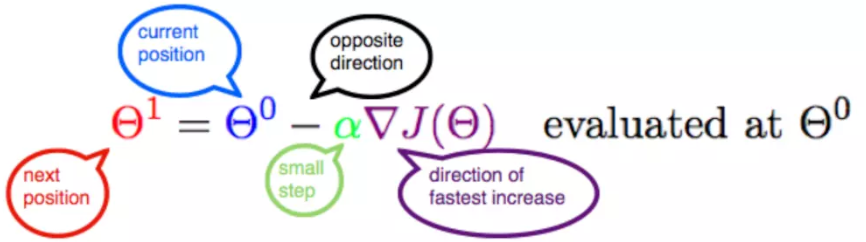2.3.1 α
α在梯度下降算法中被称作为学习率或者步长，意味着我们可以通过α来控制每一步走的距离，以保证不要步子跨的太大扯着蛋，哈哈，其实就是不要走太快，错过了最低点。同时也要保证不要走的太慢，导致太阳下山了，还没有走到山下。所以α的选择在梯度下降法中往往是很重要的！α不能太大也不能太小，太小的话，可能导致迟迟走不到最低点，太大的话，会导致错过最低点！
2.3.2 梯度要乘以一个负号
梯度前加一个负号，就意味着朝着梯度相反的方向前进！我们在前文提到，梯度的方向实际就是函数在此点上升最快的方向！而我们需要朝着下降最快的方向走，自然就是负的梯度的方向，所以此处需要加上负号；那么如果时上坡，也就是梯度上升算法，当然就不需要添加负号了。
3. 实例
我们已经基本了解了梯度下降算法的计算过程，那么我们就来看几个梯度下降算法的小实例，首先从单变量的函数开始，然后介绍多变量的函数。
3.1 单变量函数的梯度下降
我们假设有一个单变量的函数
$J(\theta) = \theta^2$
函数的微分，直接求导就可以得到
$J'(\theta) = 2\theta$
初始化，也就是起点，起点可以随意的设置，这里设置为1
$\theta^0 = 1$
学习率也可以随意的设置，这里设置为0.4
$\alpha = 0.4$
根据梯度下降的计算公式
${\color{Red} \Theta^1} = {\color{Blue} \Theta^0} + {\color{Green} \alpha} {\color{Purple} \triangledown J(\Theta)}\rightarrow evaluated at \Theta^0$
我们开始进行梯度下降的迭代计算过程：
$\theta^0 = 1$
$\theta^1 = \theta^0 - \alpha*J'(\theta^0)=1 - 0.4*2 = 0.2$
$\theta^2 = \theta^1 - \alpha*J'(\theta^1)= 0.2 - 0.4*0.4=0.04$
$\theta^3 = 0.008$
$\theta^4 = 0.0016$
如图，经过四次的运算，也就是走了四步，基本就抵达了函数的最低点，也就是山底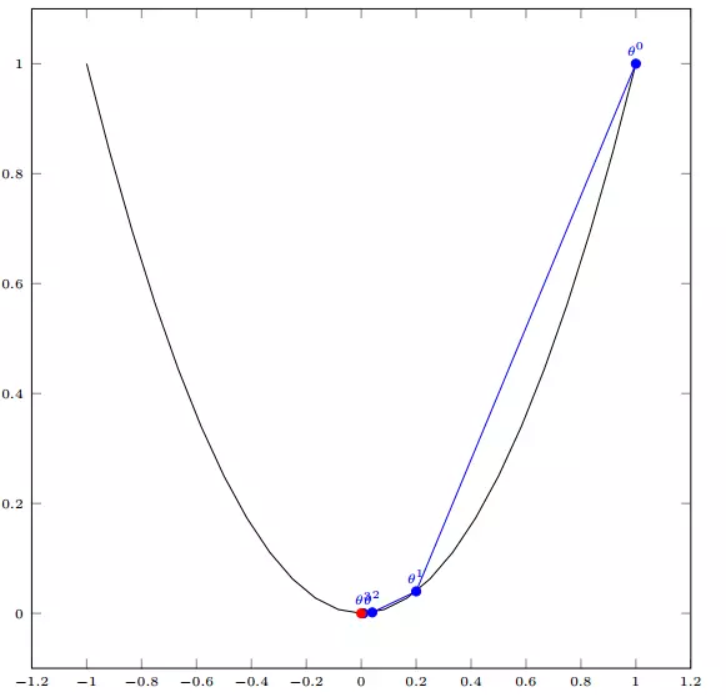3.2 多变量函数的梯度下降
我们假设有一个目标函数
$J(\Theta) = \theta_{1}^2 + \theta_{2}^2$
现在要通过梯度下降法计算这个函数的最小值。我们通过观察就能发现最小值其实就是 (0，0)点。但是接下来，我们会从梯度下降算法开始一步步计算到这个最小值！
我们假设初始的起点为：
$\Theta^0 = (1, 3)$
初始的学习率为：
$\alpha = 0.1$
函数的梯度为：
$\triangledown J(\Theta ) = \left < 2\theta_{1},2\theta_{2} \right >$
进行多次迭代：
$\Theta^0 = (1, 3)$
$\Theta^1 = \Theta^0 - \alpha\triangledown J(\Theta ) = (1,3) - 0.1*(2, 6)=(0.8, 2.4)$
$\Theta^2 = (0.8, 2.4) - 0.1*(1.6, 4.8)=(0.64, 1.92)$
$\Theta^3 =(0.5124, 1.536)$
$\Theta^4 =(0.4096, 1.228800000000001)$
$\vdots$
$\Theta^{10} =(0.1073741824000003, 0.32212254720000005)$
$\vdots$
$\Theta^{50} =(1.141798154164342e^{-05}, 3.42539442494306e^{-05})$
$\vdots$
$\Theta^{100} =(1.6296287810675902e^{-10}, 4.8888886343202771e^{-10})$
我们发现，已经基本靠近函数的最小值点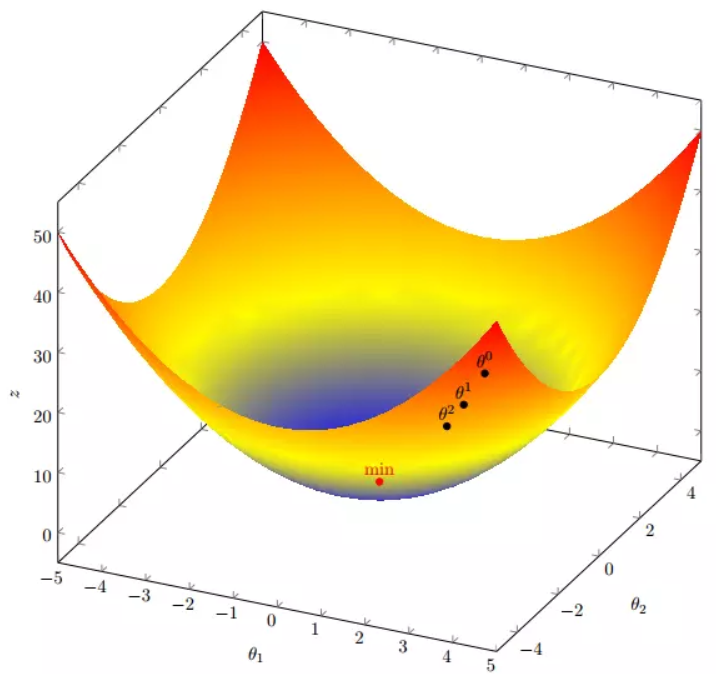4. 代码实现
4. 1 场景分析
下面我们将用python实现一个简单的梯度下降算法。场景是一个简单的线性回归的例子：假设现在我们有一系列的点，如下图所示：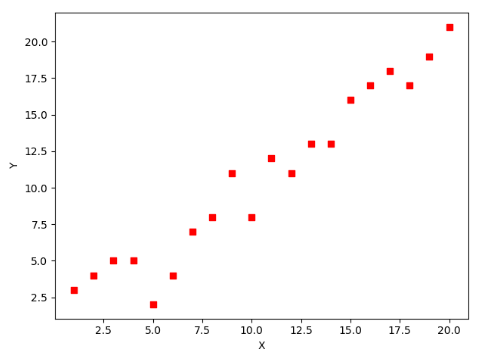我们将用梯度下降法来拟合出这条直线！
首先，我们需要定义一个代价函数，在此我们选用均方误差代价函数（也称平方误差代价函数）
$J(\Theta) = \frac{1}{2m}\sum_{i=1}^{m}(h_{\theta}(x^{(i)})-y^{(i)})^2$
此公式中

m是数据集中数据点的个数，也就是样本数
½是一个常量，这样是为了在求梯度的时候，二次方乘下来的2就和这里的½抵消了，自然就没有多余的常数系数，方便后续的计算，同时对结果不会有影响
y 是数据集中每个点的真实y坐标的值，也就是类标签
h 是我们的预测函数（假设函数），根据每一个输入x，根据Θ 计算得到预测的y值，即

$h_{\Theta}(x^{(i)}) = \Theta_{0} + \Theta_{1}x_{1}^{(i)}$
我们可以根据代价函数看到，代价函数中的变量有两个，所以是一个多变量的梯度下降问题，求解出代价函数的梯度，也就是分别对两个变量进行微分
$\triangledown J(\Theta ) = \left < \frac{\delta J}{\delta \Theta_{0}}, \frac{\delta J}{\delta \Theta_{1}} \right >$
$\frac{\delta J}{\delta \Theta_{0}} = \frac{1}{m}\sum_{i=1}^{m}(h_{\Theta}(x^{(i)})-y^{(i)})$
$\frac{\delta J}{\delta \Theta_{1}} = \frac{1}{m}\sum_{i=1}^{m}(h_{\Theta}(x^{(i)})-y^{(i)})x_{1}^{(i)}$
明确了代价函数和梯度，以及预测的函数形式。我们就可以开始编写代码了。但在这之前，需要说明一点，就是为了方便代码的编写，我们会将所有的公式都转换为矩阵的形式，python中计算矩阵是非常方便的，同时代码也会变得非常的简洁。
为了转换为矩阵的计算，我们观察到预测函数的形式
$h_{\Theta}(x^{(i)}) = \Theta_{0} + \Theta_{1}x^{(i)}$
我们有两个变量，为了对这个公式进行矩阵化，我们可以给每一个点x增加一维，这一维的值固定为1，这一维将会乘到Θ0上。这样就方便我们统一矩阵化的计算
$(x_{1}^{(i)},y^{(i)})\rightarrow (x_{0}^{(i)},x_{1}^{(i)},y^{(i)}) with x_{0}^{(i)} = 1 \forall _{i}$
然后我们将代价函数和梯度转化为矩阵向量相乘的形式
$J(\Theta) = \frac{1}{2m}(X\Theta - \vec{y})^{T}(X\Theta - \vec{y})$
$\triangledown J(\Theta) = \frac{1}{m}X^{T}(X\Theta - \vec{y}))$
4. 2 代码
首先，我们需要定义数据集和学习率
#!/usr/bin/env python3
# -*- coding: utf-8 -*-
# @Time    : 2019/1/21 21:06
# @Author  : Arrow and Bullet
# @Software: PyCharm
# @Blog    ：https://blog.csdn.net/qq_41800366

from numpy import *

# 数据集大小 即20个数据点
m = 20
# x的坐标以及对应的矩阵
X0 = ones((m, 1))  # 生成一个m行1列的向量，也就是x0，全是1
X1 = arange(1, m+1).reshape(m, 1)  # 生成一个m行1列的向量，也就是x1，从1到m
X = hstack((X0, X1))  # 按照列堆叠形成数组，其实就是样本数据
# 对应的y坐标
y = np.array([
3, 4, 5, 5, 2, 4, 7, 8, 11, 8, 12,
11, 13, 13, 16, 17, 18, 17, 19, 21
]).reshape(m, 1)
# 学习率
alpha = 0.01

接下来我们以矩阵向量的形式定义代价函数和代价函数的梯度
# 定义代价函数
def cost_function(theta, X, Y):
diff = dot(X, theta) - Y  # dot() 数组需要像矩阵那样相乘，就需要用到dot()
return (1/(2*m)) * dot(diff.transpose(), diff)

# 定义代价函数对应的梯度函数
diff = dot(X, theta) - Y
return (1/m) * dot(X.transpose(), diff)

最后就是算法的核心部分，梯度下降迭代计算
# 梯度下降迭代
theta = array([1, 1]).reshape(2, 1)
theta = theta - alpha * gradient
return theta

print('optimal:', optimal)
print('cost function:', cost_function(optimal, X, Y))

当梯度小于1e-5时，说明已经进入了比较平滑的状态，类似于山谷的状态，这时候再继续迭代效果也不大了，所以这个时候可以退出循环！
运行代码，计算得到的结果如下：
print('optimal:', optimal)  # 结果 [[0.51583286][0.96992163]]
print('cost function:', cost_function(optimal, X, Y))  # 1.014962406233101

通过matplotlib画出图像，
# 根据数据画出对应的图像
def plot(X, Y, theta):
import matplotlib.pyplot as plt
ax = plt.subplot(111)  # 这是我改的
ax.scatter(X, Y, s=30, c="red", marker="s")
plt.xlabel("X")
plt.ylabel("Y")
x = arange(0, 21, 0.2)  # x的范围
y = theta + theta*x
ax.plot(x, y)
plt.show()

plot(X1, Y, optimal)

所拟合出的直线如下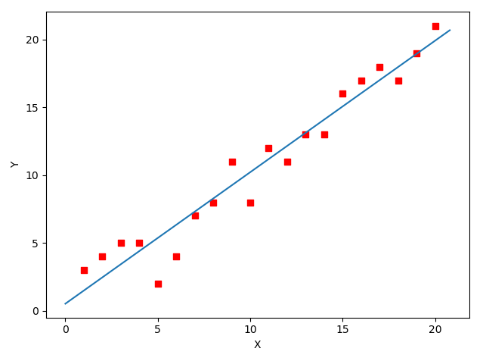全部代码如下，大家有兴趣的可以复制下来跑一下看一下结果：
#!/usr/bin/env python3
# -*- coding: utf-8 -*-
# @Time    : 2019/1/21 21:06
# @Author  : Arrow and Bullet
# @Software: PyCharm
# @Blog    ：https://blog.csdn.net/qq_41800366

from numpy import *

# 数据集大小 即20个数据点
m = 20
# x的坐标以及对应的矩阵
X0 = ones((m, 1))  # 生成一个m行1列的向量，也就是x0，全是1
X1 = arange(1, m+1).reshape(m, 1)  # 生成一个m行1列的向量，也就是x1，从1到m
X = hstack((X0, X1))  # 按照列堆叠形成数组，其实就是样本数据
# 对应的y坐标
Y = array([
3, 4, 5, 5, 2, 4, 7, 8, 11, 8, 12,
11, 13, 13, 16, 17, 18, 17, 19, 21
]).reshape(m, 1)
# 学习率
alpha = 0.01

# 定义代价函数
def cost_function(theta, X, Y):
diff = dot(X, theta) - Y  # dot() 数组需要像矩阵那样相乘，就需要用到dot()
return (1/(2*m)) * dot(diff.transpose(), diff)

# 定义代价函数对应的梯度函数
diff = dot(X, theta) - Y
return (1/m) * dot(X.transpose(), diff)

# 梯度下降迭代
theta = array([1, 1]).reshape(2, 1)
theta = theta - alpha * gradient
return theta

print('optimal:', optimal)
print('cost function:', cost_function(optimal, X, Y))

# 根据数据画出对应的图像
def plot(X, Y, theta):
import matplotlib.pyplot as plt
ax = plt.subplot(111)  # 这是我改的
ax.scatter(X, Y, s=30, c="red", marker="s")
plt.xlabel("X")
plt.ylabel("Y")
x = arange(0, 21, 0.2)  # x的范围
y = theta + theta*x
ax.plot(x, y)
plt.show()

plot(X1, Y, optimal)

5. 小结
至此，就基本介绍完了梯度下降法的基本思想和算法流程，并且用python实现了一个简单的梯度下降算法拟合直线的案例！
最后，我们回到文章开头所提出的场景假设:
这个下山的人实际上就代表了反向传播算法，下山的路径其实就代表着算法中一直在寻找的参数Θ，山上当前点的最陡峭的方向实际上就是代价函数在这一点的梯度方向，场景中观测最陡峭方向所用的工具就是微分 。在下一次观测之前的时间就是有我们算法中的学习率α所定义的。
可以看到场景假设和梯度下降算法很好的完成了对应！
本文部分内容来自一位前辈，非常感谢分享！谢谢！


展开全文Gradient Decent 梯度下降算法梯度下降算法原理平方误差代价函数进行偏微分计算不断更新参数梯度下降代码(Python)
梯度下降算法原理
本文主要简略介绍梯度下降算法的原理。梯度下降就像在一个山谷中行走，不断迈出在当前位置下降最快的那一步，直到找到一个最低点也就是收敛点，当然这个点可能是局部最优解而非全局最优解。
平方误差代价函数
我们假设有一个共包含 m 个样本的训练集 $\{x^i,y^i\},i=1,2,...,m$，x和y的关系如下图所示：我们假设 h(x)是拟合出的关于x和y关系的曲线，如果y是连续的则为回归问题，如果y是离散的则为分类问题。 函数$h(x)$如下所示，其中$\theta$为参数：
$h_\theta(x)=\theta_0+\theta_1\times x+...$
则平方误差代价函数为：
$J(\theta_0,\theta_1,...)=\frac{1}{2m} \sum^m_{i=1}(h_\theta(x^i)-y^i)^2\\ Task=minize(J)$
梯度下降算法所要做的就是将$J(\theta_0,\theta_1,...)$最小化，使假设函数$h(x)$尽可能拟合真实情况。
进行偏微分计算不断更新参数
在初始化了参数 $\theta$ 之后，接下来进行偏微分计算不断 同步更新 $\theta$，此处要注意是同步更新，不能将更新了 $\theta_0$ 后的 $J$ 代入对 $\theta_1$ 的更新计算中。假设学习速度(learning rate)为 $\alpha$，学习速度的大小选取要适当，过大容易错过收敛点，过小则需要迭代太多次，更新参数的过程如下：
$temp_j = \theta_j-\alpha\frac{\partial}{\partial \theta_j}J(\theta_0,\theta_1,...)\\ \theta_j=temp_j$
越接近局部最优解时，$\frac{\partial}{\partial \theta_j}J(\theta_0,\theta_1,...)$ 的值越小，不断重复这个更新参数的过程直至收敛，这时得到的 $h_\theta(x)$ 即为拟合效果较好的函数。
梯度下降代码(Python)
本文代码主要参考了博客梯度下降算法原理讲解——机器学习，代码编写过程中，将所有的公式都转换为矩阵的形式，python中矩阵计算比较方便，同时也会使代码更简洁。
预测函数 $h(x)$ 转化为如下形式：
$h_\Theta(x)=\Theta_0+\Theta_1\times x+...$
假设 $\theta$ 有两个，为了便于进行矩阵化，给每一个点x增加一维，这一维的值固定为1，这一维将会乘到 $\Theta_0$上。则 $x_0^i$ 与 $\Theta_0$ 相乘后仍为 $\Theta_0$，$x_1^i$ 为原来的 $x^i$。这样就方便我们统一矩阵化的计算：
$(x_1^i,y^i)->(x_0^i,x_1^i,y^i)\\ h_\Theta(x)=\Theta\times X$
代价函数和梯度（偏微分计算）转化为如下形式：
$J(\Theta)=\frac{1}{2m}( h_\Theta(x)-Y)^T( h_\Theta(x)-Y)\\ \nabla J(\Theta)=\frac{1}{m}X^T(h_\Theta(x)-Y)\\$
Python代码如下：
from numpy import *

# 数据集大小 即20个数据点
m = 20
# x的坐标以及对应的矩阵
X0 = ones((m, 1))  # 生成一个m行1列的向量，也就是x0，全是1
X1 = arange(1, m+1).reshape(m, 1)  # 生成一个m行1列的向量，也就是x1，从1到m
X = hstack((X0, X1))  # 按照列堆叠形成数组，其实就是样本数据
# 对应的y坐标
Y = array([
3, 4, 5, 5, 2, 4, 7, 8, 11, 8, 12,
11, 13, 13, 16, 17, 18, 17, 19, 21
]).reshape(m, 1) # m行1列
# 学习速度
alpha = 0.01

# 定义代价函数J
def cost_function(theta, X, Y):
# h = X*\Theta
diff = dot(X, theta) - Y  # dot() 数组需要像矩阵那样相乘，就需要用到dot()
return (1/(2*m)) * dot(diff.transpose(), diff)# h的转置和h相乘

# 定义代价函数对应的梯度函数（偏微分计算）
diff = dot(X, theta) - Y
return (1/m) * dot(X.transpose(), diff)

# 梯度下降迭代
# reshape成2行1列说明是两个\theta参数，如果\theta参数需要更多此处需要更改
theta = array([1, 1]).reshape(2, 1)
while not all(abs(gradient) <= 1e-5): # 当梯度小于1e-5时，停止迭代
theta = theta - alpha * gradient
return theta

print('optimal:', optimal)
print('cost function:', cost_function(optimal, X, Y))

# 根据数据画出对应的图像
def plot(X, Y, theta):
import matplotlib.pyplot as plt
ax = plt.subplot(111)  # 图像为一行一列分布在第一块
ax.scatter(X, Y, s=30, c="red", marker="s")
plt.xlabel("X")
plt.ylabel("Y")
x = arange(0, 21, 0.1)  # x的范围
y = theta + theta*x
ax.plot(x, y)
plt.show()

plot(X1, Y, optimal)




展开全文python
• 梯度下降算法原理视频教程，希望能够学习者提供帮助，实现对梯度下降算法基础知识的掌握与理解，为后续学习做好铺垫，实现梯度下降算法的灵活运用
• 1 原理梯度下降究竟为何方神圣？我来用最通俗的语言来介绍下：假设你站在华山之巅，你现在想以最快速度下山，那你肯定是找一条最陡峭的路走。你环顾四周，找到了一条路线，恩，这个方向是最陡的。于是你就出发了，...
今天我们就来介绍用来优化代价函数的梯度下降算法(gradient descent algorithm)。1 原理那梯度下降究竟为何方神圣？我来用最通俗的语言来介绍下：假设你站在华山之巅，你现在想以最快速度下山，那你肯定是找一条最陡峭的路走。你环顾四周，找到了一条路线，恩，这个方向是最陡的。于是你就出发了，走了一会发现，这个方向不是最陡的路了。你就停下来，换了个最陡的方向，继续往下走。重复这个步骤，你最终到达了山脚下。那么，你从山顶到山脚的整个下山的过程，就是梯度下降。为了在线性回归中应用梯度下降，我们先来回顾下线性回归模型的代价函数，它长这个样子：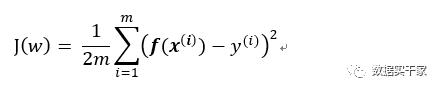其中，f(x)为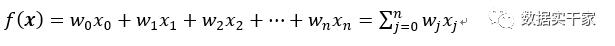注意，我们变量的上标是指样本数量，从1到m；下标指特征数量，从0到n。我们的目标是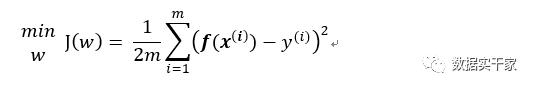即在J(w)取最小值时，所对应的w值。这时，梯度下降法就上场了，用公式表示为：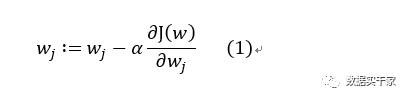其中，“:=”为赋值的含义；α为学习速率，又叫步长，可以理解为我们下山时每走一步的距离；α右边的是J(w)对w求的偏导(偏导就是对w向量中的每个元素分别求导)。这个公式的含义就是，先初始确定一个w的值，然后用(1)式计算新的w值，反复迭代。我们不断更新参数w的值，直到J(w)取得最小值时，停止迭代。我们先把(1)式中J(w)对w的偏导求出来，会容易理解些：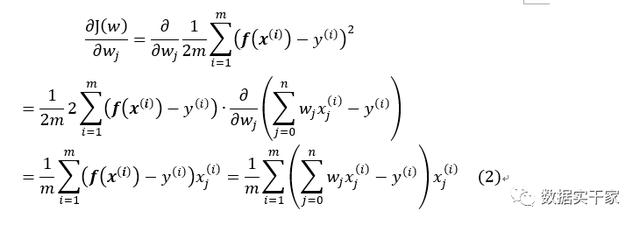将(2)式代入(1)式，可得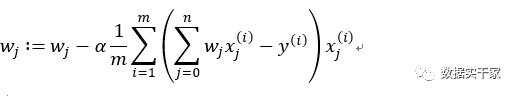这就是线性回归中的梯度下降算法。最后，我手画一张图来把梯度下降的原理大概表示下：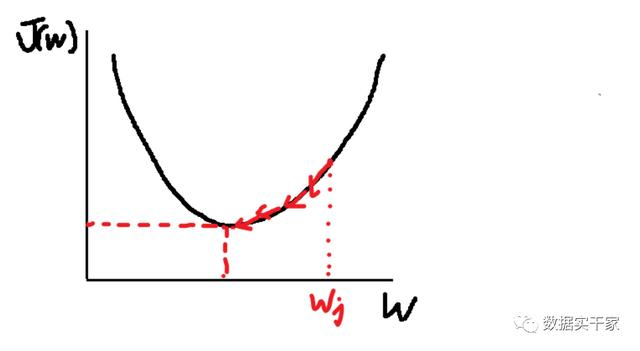如上图，我们先确定一个w，然后按步长α一步一步减小w的值。最后当w取某一值时，J(w)取得最小值，任务就完成了。说到这里，可能大家就有疑问了，梯度下降的公式(1)到底是怎么来的呢？别急，我们马上就来推导。2 推导首先，我们需要泰勒近似定理的一阶展开式：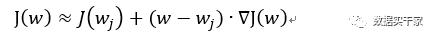上边那个倒三角符号表示梯度，就是对w求偏导的意思。从上式不难看出：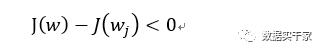也就是说：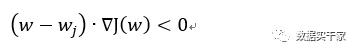上式说明了什么呢？注意到w和▽J(w)均为向量，也就是说，参数w变化的方向与梯度方向之间的夹角大于90°。我们希望J(w)每次迭代的变化量越大越好，那什么时候达到最大呢，就是参数w的变化量与梯度方向正好相反的时候，也就是二者的夹角达到180°的时候。我们用公式来说明下：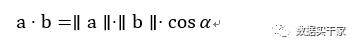也就是说，两个向量的点积等于它们的模相乘，再乘以两个向量的夹角α。不难看出，当cosα=-1时，也就是α为180°时，两个向量的点积取到负值最大。因为两个向量方向相反，故我们可推出：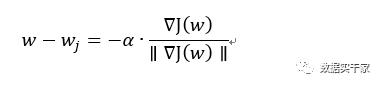其中α右边的为单位向量，可将梯度的模与阿尔法合并，简化为：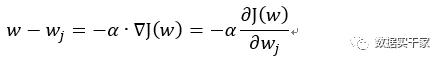移项，可得：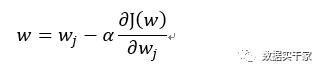这就是我们的梯度下降公式(1)，大功告成。最后我们放一张动图来看下梯度下降的效果(图片来自于网络)：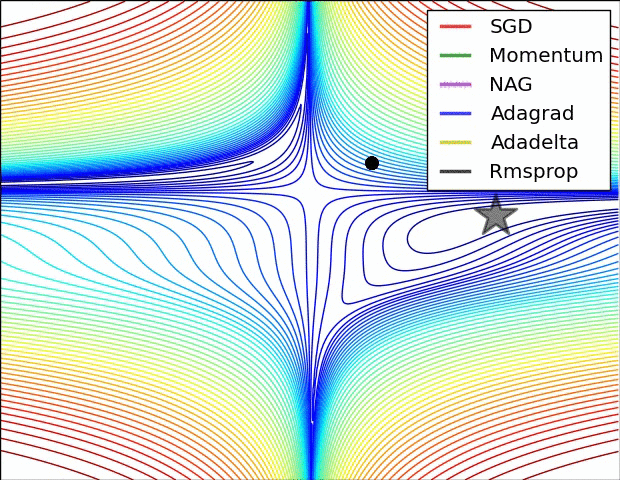下篇我会介绍有关模型拟合数据时产生的不利情况，以及如何避免这种情况发生，敬请期待。
展开全文• 求解机器学习算法的模型参数，常用两种方法：梯度下降，最小二乘法。此外还有牛顿法和拟牛顿法。...梯度下降法有代数法和矩阵法两种表示形式。 2.1 代数法 1. 先决条件：确认模型的假设函数和损失函数 线性回归...

求解机器学习算法的模型参数，常用两种方法：梯度下降，最小二乘法。此外还有牛顿法和拟牛顿法。

1. 梯度
对多元函数参数求偏导，把求得的偏导写成向量形式。比如：f(x,y)对x，y求偏导，梯度就是(∂f/∂x, ∂f/∂y)T。

2. 梯度下降法详解
梯度下降法有代数法和矩阵法两种表示形式。

2.1 代数法
1. 先决条件：确认模型的假设函数和损失函数
线性回归假设函数：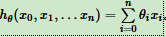线性回归损失函数：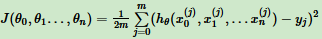2. 参数初始化
初始化θ0，θ1...θn，算法终止距离ε，步长α。一般将所有的θ初始化为0，步长为1。
3. 算法过程
(1)、求当前位置损失函数的梯度。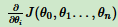(2)、步长乘以梯度，得到当前位置下降距离。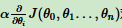(3)、确定是否所有的θi，梯度下降的距离都小于ε，如果小于ε算法终止。当前所有θi(i = 1,2...n)为最终结果。否则进入步骤4.
(4)、更新所有的θ，更新完进入步骤1.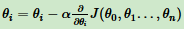线性回归的例子：
损失函数：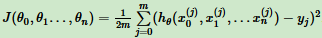按步骤1对θi求偏导：由于样本中没x0，所以上式令所有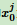为1。
步骤4中θi更新表达式为：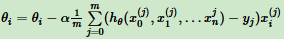注意：第3节讲梯度下降法的变种，主要区别是对样本选取的方法不同，这里采用所有样本。

2.2 矩阵法
1. 先决条件：确认模型的假设函数和损失函数
线性回归假设函数：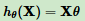线性回归损失函数：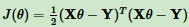2. 参数初始化
初始化θ向量为默认值，算法终止距离ε，步长α。一般将所有的θ初始化为0，步长为1。

线性回归的例子：
对θ向量求偏导数：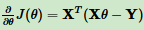θ向量的更新表达式为：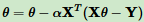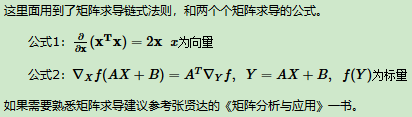2.3 梯度下降法调优
1. 步长选择。步长太大，迭代会过快，可能错过最优解；步长太小，迭代过慢。
2. 初始值选择。初始值不同，最小值可能不同，因为梯度下降法求得的是局部最小值。
3. 归一化。不同特征取值范围不一样，减少特征取值的影响，对数据归一化。
对每个特征x，求它的期望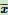和标准差std(x)，然后转化为：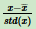这样得到新期望为0，新方差为1。

3. 梯度下降法(BGD，SGD，MBGD)
更新参数θi用所有样本进行更新。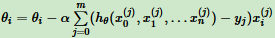更新参数θi仅用一个样本j进行更新。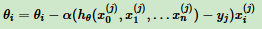小批量梯度下降法是批量梯度下降法和随机梯度下降法的折中， 对于m个样本，用x个样本来更新。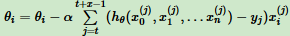来自：刘建平
hθ(x0,x1,...xn)=∑i=0nθixi
转载于:https://www.cnblogs.com/keye/p/10855497.html
展开全文• 牛顿法、梯度下降法原理及Python编程应用 一、项目概述 无论是在学习还是工作中，我们都会遇到很多最优化问题。最优化方法是一种数学方法，它是研究在给定约束之下如何寻求某些因素(的量)，以使某一(或某些)指标达到...
• 本文将以通俗易懂的方式介绍梯度下降算法原理，以及文章的最后会去简要实现梯度下降算法（python代码实现） 基本思想 首先，我们需要了解梯度下降算法原理，这里我举一个简单的例子： 有一个农夫在山上砍柴，...深度学习 机器学习
• 梯度下降算法原理笔记–机器学习 最近在看一些梯度下降的知识，看到头蒙，今天来整理一下一些相关知识，以至于后续便于理解。 机器学习中我们常见梯度下降这个名词，但是什么是梯度下降呢？梯度下降又是干嘛的呢？...机器学习
•python实现
• 梯度下降法是神经网络模型训练最常用的优化算法 对于深度学习，梯度下降法大部分的模型中都会遇到，其中也有不少学问 找到目标函数的梯度，而梯度代表的就是函数上升最快的方向 对于最小优化 哼～ ...神经网络 机器学习 人工智能 深度学习
• 梯度下降法 　1、梯度： 　在微积分里面，对多元函数参数求偏导数，把求的各参数的偏导数以向量的形式写出来，就是梯度。 　梯度向量从几何意义上讲，就是函数变化增加最快的地方，沿着梯度向量的方向更容易找到...机器学习 人工智能
• 还记得以前刚开始学习AI的时候，遇到了梯度下降算法，一直对很多概念搞不清楚，包括后来很长的一段时间也不是很明白梯度下降的实现原理，看了很多博客文章都是一知半解，总是有很多疑惑不能搞清楚，而且绝大多数的...最优化算法 机器学习机器学习 深度学习 神经网络
• 1. 概述 梯度下降（gradient descent）在机器学习中...梯度下降法的基本思想可以类比为一个下山的过程。 假设这样一个场景：一个人被困在山上，需要从山上下来(找到山的最低点，也就是山谷)。但此时山上的浓雾很大，
• 1. 概述 梯度下降（gradient descent）在机器学习中应用... 本文将从一个下山的场景开始，先提出梯度下降算法的基本思想，进而从数学上解释梯度下降算法原理，解释为什么要用梯度，最后实现一个简单的梯度下降算......